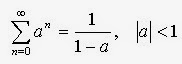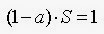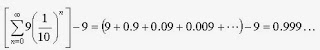## Friday, February 27, 2015

### Bad Math In Good Math

1.0 Introduction and Overview of the Book

Mark C. Chu-Carroll's blog is Good Math, Bad Math. His book is Good Math: A Geek's Guide to the Beauty of Numbers, Logic, and Computation.

A teenager recently asked me about what math he should learn if he wanted to become a computer programmer or game developer. One cannot recommend a textbook (on discrete mathematics?) to answer this, I think. If you do not mind the errors, this popular presentation will do. I like how it presents the building up of all kinds of numbers from set theory. And the order of this presentation seems right, starting with the natural numbers, but then later providing a set theoretic construction in which the Peano axioms were derived. (I suppose Chu-Carroll could also present a complementary explanation of the need for more kinds of numbers by starting out with the problem of finding roots for polynomial equations in which all coefficients are natural numbers. Eventually, you would get to the claim that an nth degree polynomial with coefficients in the complex numbers has n zeros (some possibly repeating) in the complex numbers.)

The book also has an introduction to the theory of computation, with descriptions of Finite State Machines, lambda calculus, and Turing machines. There is an outline of how the universal Turing machine cannot be improved, in terms of what functions can be computed. It doesn't help to add a second or more tapes. Nor does it help to add a two-dimensional tape. The book concludes with a presentation of a function that cannot be computed by a universal Turing machine. The halting problem, as is canonical, is used for an illustration.

2.0 Bad Math Not In Good Math

Besides being interested in popular presentations of mathematics, I was interested in seeing a book developed from blog posts. Chu-Carroll wisely leaves out a large component of his blog, namely the mocking of silly presentations of bad math. I could not do that with this blog. But there is a contrast here. The bad economics I attempt to counter is presented by supposed leaders of the field and heads of supposed good departments. The bad math Chu-Carroll usually writes about is not being to used to make the world a worse place, to obfuscate and confuse the public, to disguise critical aspects of our society. Rather, it is generally presented by people with less influence than Chu-Carroll or academic mathematicians.

2.1 Not a Proof

Anyways, I want to express some sympathy for why some might find some propositions in mathematics hard to accept. I do not want to argue such nonsense as the idea that Cantor's diagonalization argument fails, by conventional mathematical standards; that different size infinities do not exist; or that 0.999... does not equal 1. Anyways, consider the following purported proof of a theorem.

Theorem:Proof: Define S by the following:Then a S is:Subtract a S from S:Or:Thus:The above was what was to be shown.

Corollary: 0.999... = 1

Proof: First note the following:Some simple manipulations allow one to apply the theorem:Or:That is:2.2 Comments on the Non-Proof and a Valid Proof

I happen to think of the above supposed proof as a heuristic than I know yields the right answer, sort of. A student, when first presented with the above by an authority, say, in high school, might be inclined to accept it. It seems like symbols are being manipulated in conventional ways.

I do not know that I expect a student to notice how various questions are begged above. What does it mean to take an infinite sum? To multiply an infinite sum by a constant? To take the difference between two infinite sums? To define an infinitely repeating decimal number? But suppose one does ask these questions, questions whose answers are presupposed by the proof. And suppose one is vaguely aware of non-standard analysis. Besides how does inequality in the statement of the theorem arise? One might think the wool is being pulled over one's eyes.

How could one prove that 0.999... = 1? First, one might prove the following by mathematical induction:Then, after defining what it means to take a limit, one could derive the previously given formula for the infinite geometric series as a limit of the finite sum. (Notice that the restriction in the theorem follows from the proof.) Finally, the claim follows, as a corollary, as shown above.

3.0 Errata and Suggestions

I think that this is the most useful part of this post for Chu-Carroll, especially if this book goes through additional printings or editions.

• p. 7, last line: "(n + 1)(n + 2)/n" should be "(n + 1)(n + 2)/2"
• p. 11, 7 lines from bottom: "our model" should be "our axioms".
• p. 19: Associativity not listed in field axioms.
• p. 20: Since the rational numbers are a field, continuity is not part of the axioms defining a field.
• Sections 2.2 and 3.3: Does the exposition of these constructions already presume the existence of integers and real numbers, respectively?
• p. 21: Shouldn't the definition of a cut be (ignoring that this definition already assumes the existence of the real number r) something like (A, B) where:
A = {x | x rational and xr}
B = {x | x rational and x > r}
• p. 84, footnote: If one is going to note that exclusive or can be defined in terms of other operations, why not note that one of and or or can be defined in terms of the other and not? Same comment applies to if ... then.
• p. 85, last 2 lines: the line break is confusing.
• p. 95, proof by contradiction of the law of the excluded middle: Is this circular reasoning? Maybe thinking of the proof as being in a meta-language saves this, but maybe this is not the best example.
• p. 97, step 1: Unmatched left parenthesis.
• p. 106: Definition of parent is not provided, but is referenced in the text.
• p. 114, base case: Maybe this should be "partition([], [], [], []).
• p. 130: In definitions of union, intersection, and Cartesian product, logical equivalence is misprinted as some weird character. This misprinting seems to be the case throughout the book (e.g., see pp. 140, 141, and 157).
• p. 133 equation: Right arrow misprinted as ">>".
• Chapter 17: Has anybody proved ZFC consistent? I thought it was the merely the case that nobody has found an inconsistency or can see how one would come about.
• p. 148: Might mention that the order being considered in the well-ordering principle is NOT necessarily the usual, intuitive order.
• p. 148: Drop "larger" in the sentence ending as "...there's a single, unique value that is the smallest positive real number larger!"
• p. 163" "powerset" should be "power set".
• p. 164, line 6: "our choice on the continuum as an axiom" is awkward. How about, "our choice about the continuum hypothesis as an axiom"?
• p. 168, Table 3: g + d = e should be g + d = g.
• p. 171-172: Maybe list mirror symmetry or write, "in addition to mirror symmetry".
• Part VI: Can we have something on the Chomsky hierarchy?
• p. 185; p. 186, Figure 15; p. 193): Labeling state A as a final state is inconsistent with the wording on p. 185, but not the wording on p. 193. On p. 185, write "...that consist of any string containing at least one a, followed by any number of bs."
• p. 190: Would not Da(ab*) be b*, not ab*?
• p. 223: "second currying example" should be "currying example". No previous example has been presented.
• p. 225, towards bottom of page: I do not understand why α does not appear in formal definition of β.
• p. 229: Suggestion: Refer back to recursion in Section 14.2 or to chapter 18.
• p. 244, 5 lines from bottom: Probably γ should not be used here, since γ was just defined to represent Strings, not a generic type. Same comment goes for α.
• p. 245, last bullet: It seems here δ is being used for the boolean type. On the previous page, β was promised to be used for booleans, as in the first step of the example on the bottom of p. 247.
• p. 249 (Not an error): The reader is supposed to understand what "Intuitionistic logic" means, with no more background than that?
• p. 257: Are the last line of the second paragraph and the last line of the page consistent in syntax?
• Can we have an index?